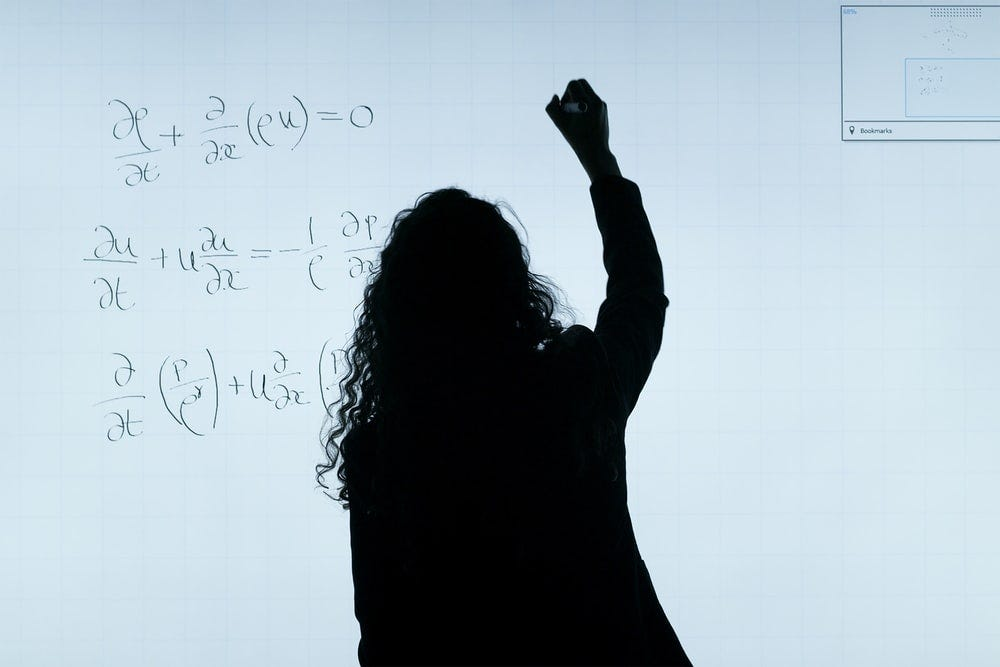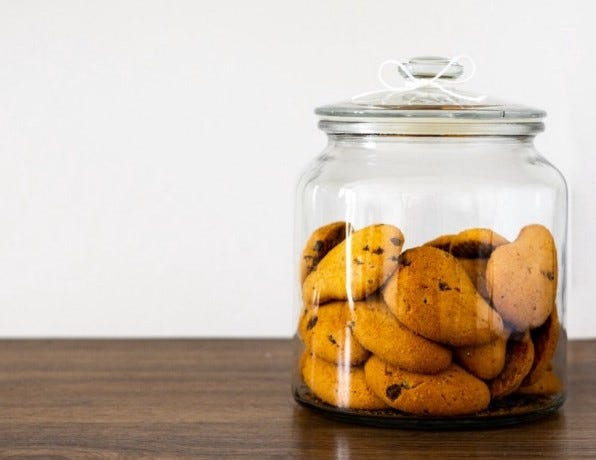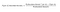I know, there is a lot of information about Bayes theorem on the internet but in my opinion, the most effective way to learn is learning by doing. I am doing elements of AI second course building AI and I was struggling with probability part. So here is a short introduction of Bayes theorem.

Hopefully, you find it useful and clear enough to understand Bayes theorem better, at least it helped me!Source

Bayes theorem is used to calculate conditional probability. Conditional probability means that we calculate the probability of event B when another event A has already happened.

Bayes theorem can be used for example to estimate what is the actual probability of having coronavirus when the test results have been positive.

# Bayes’ theorem formula

P(A|B) = the probability of A given B is true (has happened)
P(B|A) = the probability of B given A is true (has happened)
P(A) = the probability of event A
P(B) = the probability of event B

# Bayes theorem with biscuits

Let’s imagine we have two (2) jars with biscuits.Source

Jar A: 20 chocolate biscuits and 10 oat biscuits
Jar B: 20 chocolate biscuits and 20 oat biscuits

Let’s take one (1) biscuit from one of the jars, we don’t know whether it was jar A or jar B. Biscuit happens to be a chocolate biscuit.

Now let’s calculate, what is the probability that chocolate biscuit was taken from jar A.

We can calculate it by using the Bayes formula, as following:Formula to calculate what is the probability that chocolate biscuit is from jar A.

1. P(chocolate biscuit | jar A)

• The probability that the chosen jar has been a jar A when we have picked chocolate biscuit, is called likelihood
• P(chocolate biscuit | jar A) = 20 / 30 = 0,6666667
• The probability that the chosen jar is jar A in case that selected biscuit is chocolate biscuit is 67%.

2. P(jar A)

• Probability of jar A, is called the prior probability
• P(jar A) = 1 / 2 = 0,5
• The probability that we have chosen jar A is 50% because we have only two jars.

3. P(chocolate biscuits)

• The probability that selected biscuit is chocolate biscuit, is called evidence.
• P(chocolate biscuits) = 40/70 = 0,57142857
• The probability to choose chocolate biscuit is 57%.

4. P(jar A | chocolate biscuit)

• The probability that chocolate biscuit is from jar A
• P(jar A | chocolate biscuit)= (0,6667*0,5) / 0,5714 = 0,583333
• The probability that chocolate biscuit is from jar A is 58 %.

5. Controlling the result

The probability that chocolate biscuit is from jar B has to be 42%.

P(chocolate | jar B ) = 20/40 = 0,5

P(jar B) = 1/2 = 0,5

P(chocolate) = 40/70 = 0,57142857

P(jar B| chocolate biscuit) = (0,5*0,5)/0,5714= 0,4375

There is a small error due to rounding.

Thank you for reading, now you have deserved some real biscuits!

Also, thank you for the highly trained monkey correcting my text!